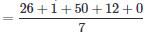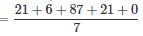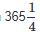Courses

# Test: Calendar 3

## 20 Questions MCQ Test Quantitative Techniques for CLAT | Test: Calendar 3

Description
This mock test of Test: Calendar 3 for Quant helps you for every Quant entrance exam. This contains 20 Multiple Choice Questions for Quant Test: Calendar 3 (mcq) to study with solutions a complete question bank. The solved questions answers in this Test: Calendar 3 quiz give you a good mix of easy questions and tough questions. Quant students definitely take this Test: Calendar 3 exercise for a better result in the exam. You can find other Test: Calendar 3 extra questions, long questions & short questions for Quant on EduRev as well by searching above.
QUESTION: 1

### What was the day of the week on 26-January-1950?

Solution:

Formula : (Date + Month code + No.of years + No.of leap year + Century code)/7=84/7
=5
=Thursday

QUESTION: 2

### Today is Thursday. What day of the week it was 30 days?

Solution:

30 days = 4 x 7 + 2 = 2 odd days
The day is 2 days before Thursday i.e Tuesday

QUESTION: 3

### 01-Jan-2007 was Monday. What day of the week lies on 01-Jan-2008?

Solution:

Given that January 1, 2007 was Monday.
Odd days in 2007 = 1 (we have taken the complete year 2007 because we need to find out the odd days from 01-Jan-2007 to 31-Dec-2007, that is the whole year 2007)
Hence January 1, 2008 = (Monday + 1 Odd day) = Tuesday

QUESTION: 4

1.12.91 is the first Sunday. Which is the fourth Tuesday of December 91?

Solution:

Given that 1.12.91 is the first Sunday
Hence we can assume that 3.12.91 is the first Tuesday
If we add 7 days to 3.12.91, we will get second Tuesday
If we add 14 days to 3.12.91, we will get third Tuesday
If we add 21 days to 3.12.91, we will get fourth Tuesday
⇒ Fourth Tuesday = (3.12.91 + 21 days) = 24.12.91

QUESTION: 5

Today is Thursday. The day after 59 days will be?

Solution:

59 days = 8 weeks 3 days = 3 odd days
Hence if today is Thursday, After 59 days, it will be
= (Thursday + 3 odd days)
= Sunday

QUESTION: 6

What was day of the week on 21-September-1987?

Solution:

Formula : (Date + Month code + No.of years + No.of leap year + Century code)/7=135/7
=2
=Monday

QUESTION: 7

How many odd days are there from 13th May, 2005 to 19th August 2005 (both inclusive)?

Solution:

Here we have to count the number days from 13th May, 2005 to 18rd August 2005 ( both inclusive)
From 13th to 31st May = 19 days
In June = 30 days
In July = 31 days
From 1st to 19th April = 19 days
Total number of days = 19 + 30 + 31 + 19 = 99 days
The number of odd days are = 14 x 7 + 1 = 99
So there is 1 odd day in the given period

QUESTION: 8

What is the year next to 1990 which will have the same calendar as that of the year 1990?

Solution:

For a year to have the same calendar with 1990 ,total odd days from 1990 should be 0. Take the year 1992 from the given choices.
Total odd days in the period 1990-1991 = 2 normal years
⇒ 2 x 1 = 2 odd daysTake the year 1995 from the given choices.
Number of odd days in the period 1990-1994 = 4 normal years + 1 leap year
⇒ 4 x 1 + 1 x 2 = 6 odd daysTake the year 1996 from the given choices.
Number of odd days in the period 1990-1995 = 5 normal years + 1 leap year
⇒ 5 x 1 + 1 x 2 = 7 odd days = 0 odd days
(As we can reduce multiples of 7 from odd days which will not change anything) Though number of odd days in the period 1990-1995 is 0, there is a catch here.
1990 is not a leap year whereas 1996 is a leap year.
Hence calendar for 1990 and 1996 will never be the same.Take the year 2001 from the given choices. Number of odd days in the period 1990-2000 = 8 normal years + 3 leap years
⇒ 8 x 1 + 3 x 2 = 14 odd days = 0 odd days
Also, both 1990 and 2001 are normal years.
Hence 1990 will have the same calendar as that of 2001

QUESTION: 9

Find the number of odd days in 126 years.

Solution:

A period of 100 years has 5 odd days . In 26 years , 4 are leap, remaining are ordinary years
125 years = 100 years + 26 years
= 100 years + 6 leap years + 20 ordinary years
= 5 odd days + 12 odd days + 20 odd days
= 37 odd days = 5 x 7 +2 = 2 odd days

QUESTION: 10

If the day before yesterday was Thursday, when will Sunday be?

Solution:

Day before yesterday was Thursday
⇒ Yesterday was a Friday
⇒ Today is a Saturday
⇒ Tomorrow is a Sunday

QUESTION: 11

How many days are there from 3rd February, 2012 to 18th April 2012 (both inclusive)?

Solution:

Here we have to count the number days from 3rd February, 2012 to 18th April 2012 ( both inclusive)
The given year is leap year, So February month has 29 days
From 3rd to 29th February = 27 days
In March = 31 days
From 1st to 18th April = 18 days
Total number of days = 27 + 31 + 18 = 76 days

QUESTION: 12

What was the day on 15th august 1947 ?

Solution:

15th Aug, 1947 = (1946 years + Period from 1.1.1947 to 15.8.1947)
Odd days in 1600 years = 0
Odd days in 300 years = 1
46 years
= (35 ordinary years + 11 leap years)
= (35 x 1 + 11 x 2)
= 57 (8 weeks + 1 day)
= 1 odd day
Jan.   Feb.   Mar.   Apr.   May.   Jun.   Jul.   Aug
( 31 + 28 + 31 + 30 + 31 + 30 + 31 + 15 )
= 227 days
= (32 weeks + 3 days)
= 3 odd days
Total number of odd days = (0 + 1 + 1 + 3) = 5 odd days
Hence, as the number of odd days = 5, given day is Friday.

QUESTION: 13

What was the day of the week on, 16th July, 1776?

Solution:

16th July, 1776 = (1775 years + Period from 1st Jan, 1776 to 16th July, 1776)
Counting of odd days :
1600 years have 0 odd day
100 years have 5 odd days
75 years = (18 leap years + 57 ordinary years)
= [(18 x 2) + (57 x 1)]
= 93 (13 weeks + 2 days)
= 2 odd days
1775 years have (0 + 5 + 2) odd days = 7 odd days = 0 odd day
Jan   Feb   Mar   Apr   May   Jun   Jul
31 + 29 + 31 + 30 + 31 + 30 + 16
= 198 days
= (28 weeks + 2 days)
Total number of odd days = (0 + 2) = 2
Required day was 'Tuesday'.

QUESTION: 14

The maximum gap between two successive leap year is?

Solution:

This can be illustrated with an example.
Ex: 1896 is a leap year.The next leap year comes in 1904 (1900 is not a leap year).
Explanation : The length of the solar year, however, is slightly less thandays-by about 11 minutes. To compensate for this discrepancy, the leap year is omitted three times every four hundred years.
In other words, a century year cannot be a leap year unless it is divisible by 400. Thus 1700, 1800, and 1900 were not leap years, but 1600, 2000, and 2400 are leap years.

QUESTION: 15

How many leap years does 100 years have?

Solution:

Given year is divided by 4, and the quotient gives the number of leap years.
Here, 100%4 = 25
But, as 100 is not a leap year ⇒ 25 - 1 = 24 leap years.

QUESTION: 16

Which two months in a year have the same calendar?

Solution:

If the period between the two months is divisible by 7, then that two months will have the same calendar.
(a). Oct + Nov = 31 + 30 = 61 (not divisible by 7)
(b). Apr + May + Jun + Jul + Aug + Sep + Oct = 30 + 31 + 30 + 31 + 31 + 30 + 31 = 214 (not divisible by 7)
(c). Jun + July + Aug + Sep = 30 + 31 + 31 + 30 = 122 (not divisible by 7)
(d). Apr + May + June = 30 + 31 + 30 = 91 (divisible by 7)
Hence, April and July months will have the same calendar.

QUESTION: 17

How many leap years do 300 years have?

Solution:

Given year is divided by 4, and the quotient gives the number of leap years.
Here, 300%4 = 75.
But, as 100, 200 and 300 are not leap years ⇒ 75 - 3 = 72 leap years.

QUESTION: 18

On what dates of July. 2004 did Monday fall?

Solution:

Let us find the day on 1st July, 2004.
2000 years have 0 odd day. 3 ordinary years have 3 odd days.
Jan.   Feb.   March   April   May   June   July
31 + 29 + 31 + 30 + 31 + 30 + 1
= 183 days
= (26 weeks + 1 day)
Total number of odd days = (0 + 3 + 1) odd days = 4 odd days.
∴ 1st July 2004 was 'Thursday'
Thus, 1st Monday in July 2004 as on 5th July. Hence, during July 2004, Monday fell on 5th, 12th, 19th and 26th.

QUESTION: 19

The year next to 2005 will have the same calendar as that of the year 2005?

Solution:

NOTE :
Repetition of leap year ⇒ Add + 28 to the Given Year.
Repetition of non leap year
Step 1 : Add + 11 to the Given Year. If Result is a leap year, Go to step 2.
Step 2: Add + 6 to the Given Year.

Solution :
Given Year is 2005, Which is a non leap year.
Step 1 : Add + 11 to the given year (i.e 2005 + 11) = 2016, Which is a leap year.
Step 2 : Add + 6 to the given year (i.e 2005 + 6) = 2011
Therefore, The calendar for the year 2005 will be same for the year 2011

QUESTION: 20

If Feb 12th,1986 falls on Wednesday then Jan 1st,1987 falls on which day?

Solution:

First,we count the number of odd days for the left over days in the given period.
Here,given period is 12.2.1986 to 1.1.1987
Feb   Mar   Apr   May   June   July   Aug   Sept   Oct   Nov   Dec   Jan
16   31   30   31   30   31   31   30   31   30   31   1 (left days)
2 + 3 + 2 + 3 + 2 + 3 + 3 + 2 + 3 + 2 + 3 + 1(odd days)
= 1 odd day
So, given day Wednesday + 1 = Thursday is the required result.